Courses

# Force and Laws of Motion Class 9 Notes | EduRev

## Class 9 : Force and Laws of Motion Class 9 Notes | EduRev

The document Force and Laws of Motion Class 9 Notes | EduRev is a part of Class 9 category.
All you need of Class 9 at this link: Class 9

Force

• A force is a push or pull upon an object resulting from the object's interaction with another object.
• Whenever there is an interaction between two objects, there is a force upon each of the objects.
•  When the interaction ceases, the two objects no longer experience the force.
• Force is a quantity that is measured using the standard metric unit known as the Newton. A Newton is abbreviated by an "N." To say "10.0 N" means 10.0 Newton of force.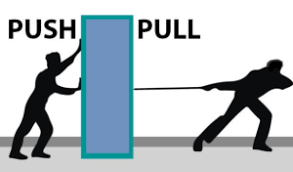Force

»Types of Forces

For simplicity sake, all forces (interactions) between objects can be placed into two broad categories:

1. contact forces,
2. forces resulting from action-at-a-distance

Contact forces are those types of forces that result when the two interacting objects are perceived to be physically contacting each other.

Examples: Frictional forces, tensional forces, normal forces, air resistance forces, and applied forces.

Action-at-a-distance forces are those types of forces that result even when the two interacting objects are not in physical contact with each other, yet are able to exert a push or pull despite their physical separation.

Examples: Gravitational forces, Magnetic forces, Electromagnetic forces, etc.

Try yourself:Which of the following is a contact force?

»Balanced and Unbalanced Forces

• When balanced forces are applied to an object, there will be no net effective force acting on the object. Balanced forces do not cause a change in motion.
• Unbalanced forces acting on an object change its speed and/or direction of motion. It moves in the direction of the force with the highest magnitude.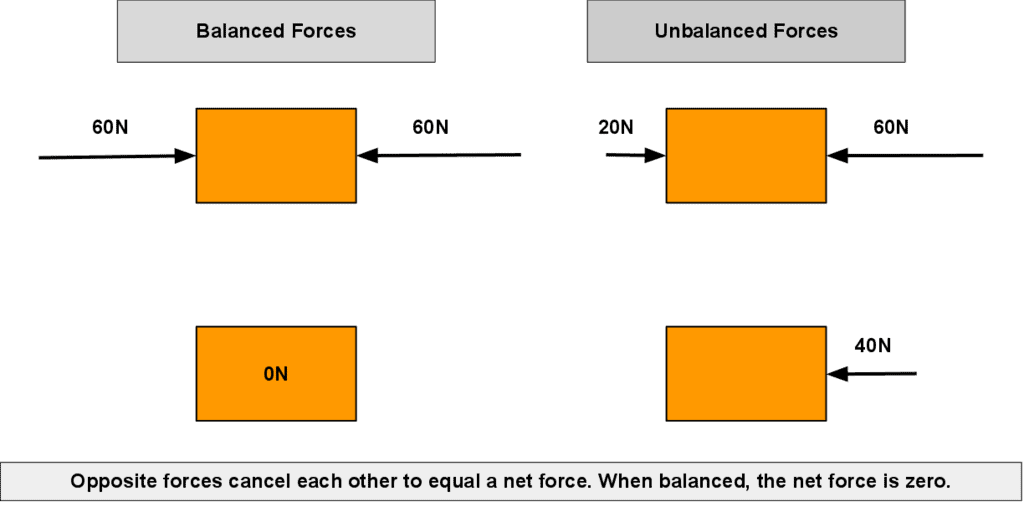Balanced and Unbalanced Forces

Laws of motion

Laws of motion, relations between the forces acting on a body and the motion of the body, first formulated by English physicist and mathematician Sir Isaac Newton. He studied the ideas of Galileo and gave three laws of motion.

1. First Law of Motion

A body continues to be in the state of rest or uniform motion in a straight line unless acted upon by an external unbalanced force. The First Law is also called the Law of Inertia.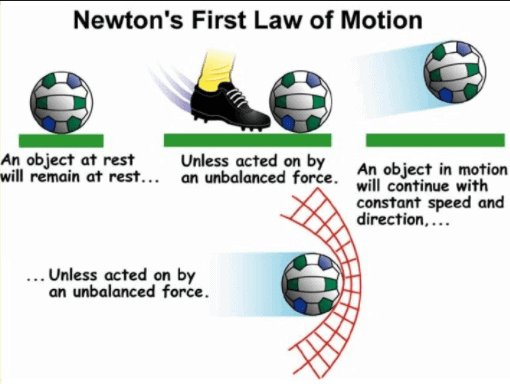» Inertia

Basically, all objects have a tendency to resist the change in the state of motion or rest. This tendency is called inertia. All bodies do not have the same inertia. Inertia depends on the mass of a body. The mass of an object is the measure of its inertia. More the mass, more the inertia, and vice-versa.

2. Second Law of Motion

» Momentum

Impacts produced by objects depend on their mass and velocity. The momentum of an object is defined as the product of its mass and velocity. p = mv. Vector quantity, has direction and magnitude.

» Second Law of Motion

The rate of change of momentum of an object is directly proportional to the applied unbalanced force in the direction of the force.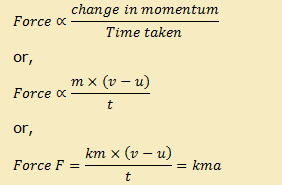For 1 unit of force on 1 kg mass with the acceleration of 1m/s2, the value of k = 1.

Therefore, F = ma.

3. Third Law of Motion

Newton’s 3rd law states that every action has an equal and opposite reaction. Action and reaction forces are equal, opposite, and acting on different bodies.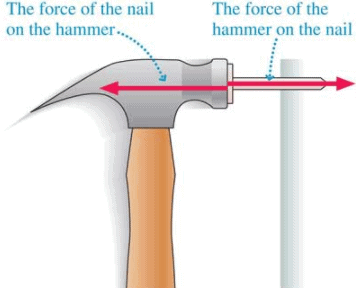Offer running on EduRev: Apply code STAYHOME200 to get INR 200 off on our premium plan EduRev Infinity!

,

,

,

,

,

,

,

,

,

,

,

,

,

,

,

,

,

,

,

,

,

;